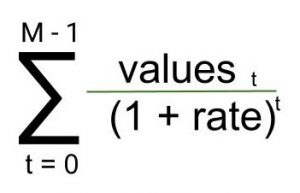# numpy.npv() in Python

• Last Updated : 17 May, 2022

numpy.npv(rate, value) : This financial function helps user to calculate the NPV(Net Present Value) of a cash flow series.

Parameters :

```rate  : [scalar] Rate of discount
value : [array_like, shape(M,)] value of
cash flows time series. The (fixed) time interval
between cash flow “events” must be the same as that
for given rate is given. By convention, investments
or “deposits” are -ve, income or “withdrawals” are +ve```

Return :

`present value as per given parameters.`

Equation being solved :Code 1 : Working

## Python

 `## Python program explaining npv() function``import` `numpy as np` `#            rate            values    ``a ``=`  `np.npv(``0.281``,[``-``100``, ``19``, ``49``, ``58``, ``200``])``print``("Net Present Value(npv) : ", a)`

Output :

`Net Present Value(npv) :  46.5579792365`
My Personal Notes arrow_drop_up